# 反馈型ANC系统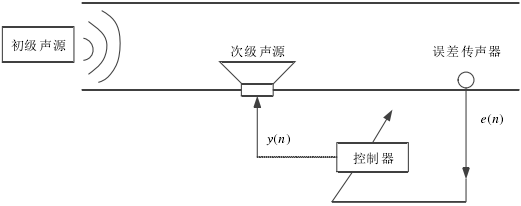反馈型ANC系统避免了对初级噪声的提取，也就不存在FM问题，反馈系统因具有一定的主动阻尼而可以有效抑制系统的暂态信号。但是反馈型系统鲁棒性较差，且对宽带噪声的处理能力较差，一般适用于无法安装参考信号传感器的场景。

# 前馈型ANC系统

前馈型ANC系统相较于反馈型增加了一个获取参考信号的传感器。误差传感器测得的残余噪声信号连同传感器获取的参考信号均作为控制器的输入。参考信号可由声学传感器获取，也可由非声学传感器（如转速计、加速度计等）获得。但声学传感器的引入容易造成声反馈现象，从而削弱系统的鲁棒性。故而窄带 ANC 系统中的参考信号一般由非声学传感器采集。通过非声学传感器可采集到噪声源信号的同步信号（如转速、加速度等），按照一定的线性关系可将其转换为频率值，根据该频率值可使信号发生器产生与窄带噪声频率一致的参考信号。前馈型 ANC 系统的物理模型如图 2.2 所示。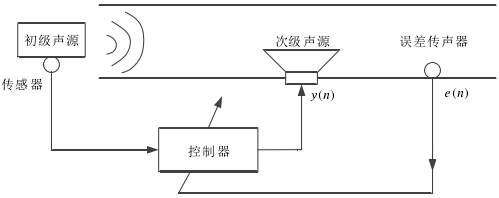其中，控制器以参考信号和残余噪声为输入，产生并调节次级声源信号$y_n$ ，驱动次级扬声器发出次级噪声，与初级声源产生的噪声进行相消干涉，最终使得误差传感器处的声压值最小。前馈型 ANC 系统具有很强的鲁棒性，且不仅适用于窄带噪声信号，也可用于处理宽带噪声信号。

# 传统ANC算法

噪声信号随时间的推移而不断变化，其特性无法进行预先估计，这一性质导致噪声很难被实时跟踪。ANC 技术要求对时变的噪声输入信号进行跟踪，从而调节控制器使产生的次级噪声信号能最大的削弱噪声输入信号自适应数字信号处理可以很好的跟踪时变信号，通过某种优化误差准则不断调整产生所需信号。而这种优化误差准则实际上就属于 ANC 算法。

## FXLMS算法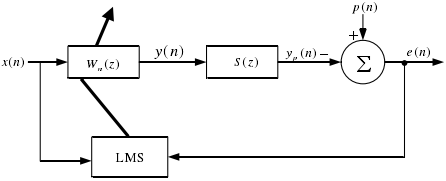ANC系统传统算法结构

其中$W_n(z)$为自适应滤波器，$S(z)$次级通道；$p(n)$为初级噪声；$e(n)$为系统残余噪声；x(n)为参考噪声。最小均方误差(Least Mean Square, LMS)算法以最陡下降法为奠基石，具有较为出众的迭代更新速度，应用非常广泛。但在ANC系统中，次级通道$S(z)$的存在会导致残余信号和参考信号间存在延时，进而削弱ANC系统的稳定性。

Morgan 在 LMS 的基础上提出了 FXLMS，在参考信号$x(n)$送入 LMS 算法模块前增加一个对次级通道传递函数的估计$S(z)$，从而解决次级通道所引起的相关延时问题，如图 2.4 所示。FXLMS 算法应用在 ANC 系统中简单且有效，在 ANC 算法中可谓历久而弥新，得到了广泛推广。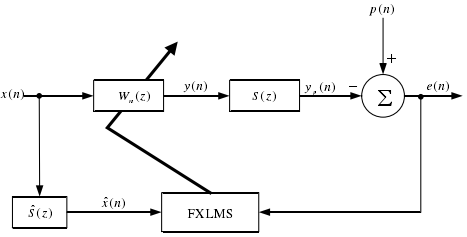FXLMS算法结构

## 次级通道在线辨识

在ANC系统中，通常将数模转换(D\A)、信号方法电路、模数转换（A\D）、滤波电路、扬声器、传声器等电子设备以及扬声器到传声器之间的实际管道等物理通道的组合称之为次级通道。就实际应用而言，次级通道的传递函数显然无法明确得知，而 ANC 系统中的自适应滤波器可对次级通道进行估计。当传递函数为时变函数时，采用在线辨识算法，即在 ANC 系统运行的同时对次级通道进行估计。下图给出了含在线辨识的 FXLMS 算法结构，自适应滤波器的输出$y_n$引入线辨识系统，作为LMS 算法的输入。同时将系统中的残余噪声信号与$y_n$经次级通道的估计之后得到的$\hat{y}_p(n)$相比较，将所得到的误差也用作 LMS 算法的激励，调节参数，不断逼近次级通道传递函数，完成对次级通道的实时在线辨识。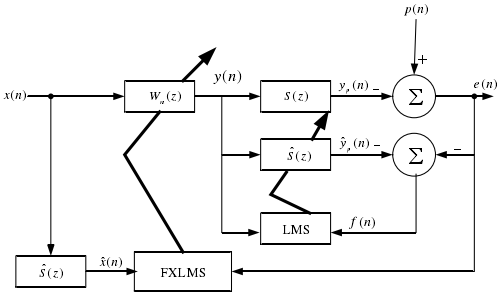次级通道的在线辨识应当满足两个基本要求：实现对次级通道较为精准的估计；同时在线辨识不应该对主降噪系统产生影响。而图 2.5 所示的次级通道的在线辨识对$y(n)$进行直接处理，使得这两个基本要求相互矛盾。故而实际的 ANC 系统应在在线辨识不被干扰与辨识不对主降噪系统产生干扰之间取得一个相对平衡。为在这两者间获取更为适宜的平衡点，Eriksson 提出增加辅助随机噪声作为在线辨识系统的激励，而在主降噪系统中$y(n)$减去辅助噪声之后再驱动扬声器产生声音信号进入次级通道。但辅助噪声又对残余噪声造成了影响，Lan 在研究宽带 ANC系统时提出了通过$|e(n)|$对辅助噪声进行进一步的约束。刘在Lan 的基础上针对窄带前馈 ANC系统，改为利用$|e(n-1)|$对送入系统的辅助噪声进行约束，如图2.6 所示。本文所展开的在线辨识采用的正是该方法。

次级通道的在线辨识可对时变次级通道传递函数进行估计，但同时增加了整个 ANC 系统的负担，此外，辅助噪声的存在仍在一定程度上削弱了 ANC 系统的鲁棒性。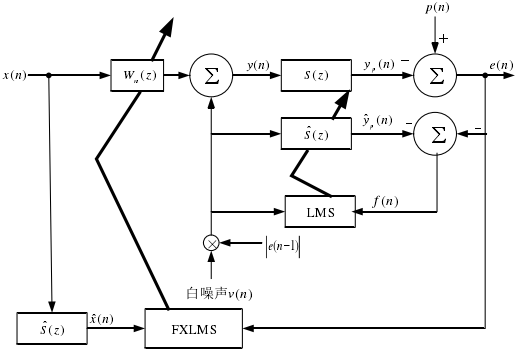## 次级通道离线辨识

当次级通道环境不随着时间而改变时，可以使用离线辨识算法。离线辨识是运行 ANC 系统进行降噪之前针对次级通道预先进行训练估计，固定并保存作为训练结果的次级通道模型，再将该模型引入到 ANC 系统并启动系统进行降噪。次级通道的离线辨识原理图如图 2.7 所示。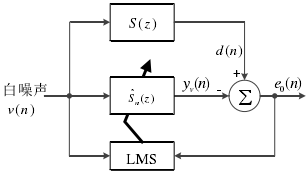其中，白噪声信号$v(n)$作为系统在第n 时刻的激励，此刻次级通道的输出为$d(n)$，即离线辨识系统的期望信号。$y_v(n)$则是白噪声激励信号经过次级通道的估计函数$\hat{S}_n(z)$的输出，$y_v(n)$与期望信号$d(n)$之差即为辨识误差$e_o(n)$，送入到 LMS 算法中。经过不断迭代更新后当辨识误差$e_o(n)$趋近于零时，$y_v(n)$与期望信号$d(n)$趋近于相同，则可知次级通道的估计函数$\hat{S}_n(z)$趋近于次级通道$S(z)$，实现了对次级通道的离线辨识。

离线辨识是脱离 ANC 降噪系统，独立进行系统辨识，不会给 ANC 降噪系统增加运行负担，同样不会损害 ANC 降噪系统的鲁棒性。若 ANC 系统的次级通道传递函数时不变，则离线辨识具有一定的优势，在本文后期具体实验时会给出更为直观的说明。

# FM 问题及非平稳

在前馈型 ANC 系统中，对参考信号的提取采用非声学传感器时，若传感器长时间工作将会累积损耗最终致使精度减削，采集到的参考信号频率将与实际初级噪声的频率存在误差。又或者，当信号发生器发出的信号不够精确，与期望存在误差。这些情况最终导致参考信号频率与实际噪声频率间存在误差，即所谓的 FM。

FM 问题对窄带前馈 ANC 具有致命性的影响力。哪怕系统中仅存在 1%的 FM 量，也将导致系统无法进行有效消噪。本文将在第三章和第六章分别从实时仿真以及实际实验两方面分析说明 FM 问题。

另外，噪声源设备的速度变化将直接表现为初级噪声信号频率的变化，而频率不稳定的初级噪声对系统的鲁棒性及跟踪能力有着毁坏性的损伤。非平稳一直是存在于实际的 ANC 应用中不可避免的又一问题，当初级噪声表现出非平稳时，采集参考信号的传感器会有响应延时并最终引起非平稳的 FM。在本文的第三章将对非平稳的 FM 进行仿真分析。

# 本章小结

本章对 ANC 技术的理论基础进行了详细介绍。首先，针对 ANC 系统分别从反馈型和前馈型两种类型对 ANC 系统类型进行概要描述。其次，详细描述了传统 ANC 算法，对 FXLMS 算法的基础 LMS 略有介绍，重点以 FXLMS 算法展开论述，继而详解了次级通道的在线辨识与离线辨识，并对在线辨识与离线辨识的应用场景进行了简要说明。最后，针对存在于前馈型 ANC系统中的 FM 及非平稳问题进行简要说明。x(n)：参考信号

u(n)：控制信号

d(n)：期望信号

y(n)：输出信号

r(n)：x滤波后的信号

e(n)：误差信号

W(z)：自适应滤波器

G(z)：真实的次级通道

$\hat{G}(z)$：估计的次级通道

%              +-----------+                       +
% x(k) ---+--->|   P(z)    |--yp(k)----------------> sum --+---> e(k)
%         |    +-----------+                          ^-   |
%         |                                           |    |
%         |        \                                ys(k)  |
%         |    +-----------+          +-----------+   |    |
%         +--->|   C(z)    |--yw(k)-->|   S(z)    |---+    |
%         |    +-----------+          +-----------+        |
%         |            \                                   |
%         |             \----------------\                 |
%         |                               \                |
%         |    +-----------+          +-----------+        |
%         +--->|   Sh(z)   |--xs(k)-->|    LMS    |<-------+
%              +-----------+          +-----------+

% LMS最小均方误差
% S(z)次级通道传递函数      % ys(k)次级声源
% P(z)主通道传递函数        % yp(k)初级声源
% C(z)控制器               % yw(k)控制器
% Sh(z)传感器函数          % xs(k)传感器参考信号

clear
T=1000; % 仿真持续时间

% 我们不知道p(z)和S(z)，所以我们必须建立dummy虚拟路径
Pw=[0.01 0.25 0.5 1 0.5 0.25 0.01];
Sw=Pw*0.25;

x_iden=randn(1,T); % 产生shape=(1,1000)的白噪声信号估计S(z)

% 送至actuator执行，在传感器位置测量，
y_iden=filter(Sw, 1, x_iden);

% 然后，开始识别过程
Shx=zeros(1,16);       % 传感器Sh(z)的状态
Shw=zeros(1,16);       % 传感器Sh(z)的权重
e_iden=zeros(1,  T);   % 识别错误的数据缓冲区

%LMS 算法
% [Shy,Shw]=lms(Shx,y_iden,x_iden,Shw,e_iden,T);
mu=0.1;                         % 学习率
for k=1:T                      % 离散时间 k
Shx=[x_iden(k) Shx(1:15)];  % 更新传感器的状态
Shy=sum(Shx.*Shw);            % 计算传感器Sh(z)的输出
e_iden(k)=y_iden(k)-Shy;    % 计算误差
Shw=Shw+mu*e_iden(k)*Shx;   % 调整权重
end

% 检查结果
subplot(2,1,1)
plot((1:T), e_iden)
ylabel('Amplitude');
xlabel('Discrete time k');
legend('Identification error');
subplot(2,1,2)
stem(Sw)
hold on
stem(Shw, 'r*')
ylabel('Amplitude');
xlabel('Numbering of filter tap');
legend('S(z)系数', 'Sh(z)系数')

X=randn(1,T);

% 测量传感器位置接收的噪声，
Yd=filter(Pw, 1, X);

% 启动系统
Cx=zeros(1,16);       % C(z)的状态
Cw=zeros(1,16);       % C(z)的权重
Sx=zeros(size(Sw));   % secondary次路径的虚拟状态
e_cont=zeros(1,T);    % 控制错误的数据缓冲区
Xhx=zeros(1,16);      % 过滤后x(k)的状态

% FxLMS 算法
% [Cy,Cw]=FxLMS(X,Cx,Cw,Sx,Sw,Shx,Shw,e_cont,Xhx,T,Yd);
mu=0.1;                            % 学习率
for k=1:T                          % 离散时间 k
Cx=[X(k) Cx(1:15)];            % 更新控制器状态
Cy=sum(Cx.*Cw);                % 计算控制器输出
Sx=[Cy Sx(1:length(Sx)-1)];    % 传播到secondary path
e_cont(k)=Yd(k)-sum(Sx.*Sw);   % 测量残差
Shx=[X(k) Shx(1:15)];          % 更新Sh(z)的状态
Xhx=[sum(Shx.*Shw) Xhx(1:15)]; % 计算过滤后的x(k)
Cw=Cw+mu*e_cont(k)*Xhx;        % 调整controller的权重
end

% 结果
figure
subplot(2,1,1)
plot((1:T), e_cont)
ylabel('Amplitude');
xlabel('Discrete time k');
legend('Noise residue')
subplot(2,1,2)
plot((1:T), Yd)
hold on
plot((1:T), Yd-e_cont, 'r:')
ylabel('Amplitude');
xlabel('Discrete time k');
legend('噪声信号', '控制信号')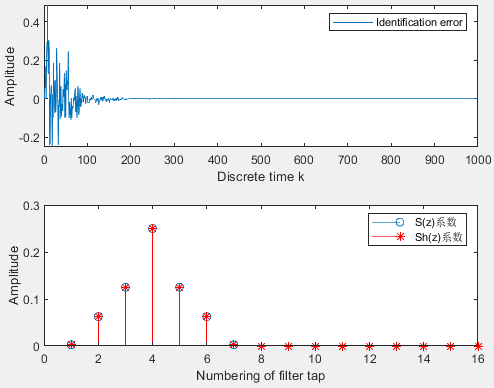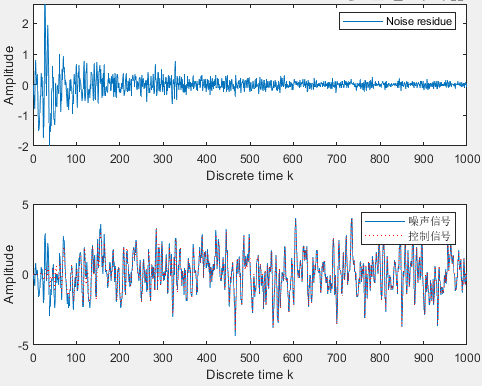# 参考文献

posted @ 2019-10-18 10:14  凌逆战  阅读(...)  评论(... 编辑 收藏